Ramanujan's tau function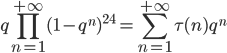It looks simple, isn't it ?
But computation of τ(n) is not ...

First values are: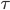(1) = 1(2) = -24(3) = 252(4) = -1472(5) = 4830(6) = -6048

Ramanujan found it has remarkable properties: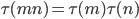for m, n coprime integers,for p prime.

Now we need a formula to compute τ(p) for all primes p.

A formula related to Catalan triangle

From Eichler-Selberg trace formula, one may derive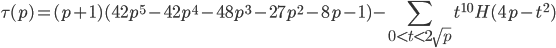where p is prime, and H(n) is Hurwitz-Kronecker class number of binary quadratic forms of negative discriminant -n.

A new and faster formula

Recombining several traces leads to the formula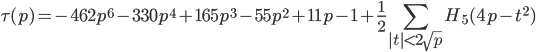where p is prime and H5(n)=n5H(n).

Note that it mainly consists of a finite sum in the table of H5(n) integers. Hence it is a somewhat faster algorithm when computing τ(p) for all primes.

After rearrangement, I obtained the nice formulation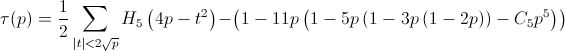where C5 = 42 is the 5th Catalan number.

Non-ordinary primes

We found that the only primes p for which τ(p) ≡ 0 (mod p)  are 2, 3, 5, 7, 2411 and 7758337633, up to 1010.

See OEIS entry: A007659

Odd prime values

Our purpose is to identify the integers n for which τ(n) is an odd prime, disregarding the sign of τ(n). The main result is the following :

Theorem
Let n be a positive integer such that τ(n) is an odd prime. Then n is of the form pq-1 where p and q are odd primes and p is ordinary.

One sets LR(p, q) := τ(pq-1), where "LR" stands for Lehmer-Ramanujan.

Here we give all known pairs (p,q), p < 100, such that LR(p, q) is prime (P) or probable prime (PRP):

 (p, q) Digits* Primality Verified by Software End date Duration (11, 317) 1810 P Olivier Rozier PARI/GP (17, 433) 2924 P Olivier Rozier PARI/GP 12/02/2011 10 hrs (29, 31) 242 P (29, 83) 660 P (29, 229) 1834 P Olivier Rozier PARI/GP (41, 2297) 20367 P François Morain ECPP 06/04/2018 24 days (41, 28289) 250924 PRP (47, 5) 37 P (47, 47) 424 P (47, 4177) 38404 P Andreas Enge CM/ECPP 22/06/2022 15 days (59, 1381) 13441 P Gérasimos Politis Primo 12/10/2013 83 days (59, 8971) 87365 PRP (79, 1571) 16386 P Gérasimos Politis Primo 07/02/2014 (79, 6317) 65920 PRP (89, 73) 772 P (97, 331) 3606 P Olivier Rozier PARI/GP 16/02/2011 31 hrs (97, 887) 9682 P Keira Stambolidou Primo 26/05/2013
(*) number of decimal digits of LR(p, q)

and the distribution of such values, for p < 1000, on a semi-log scale :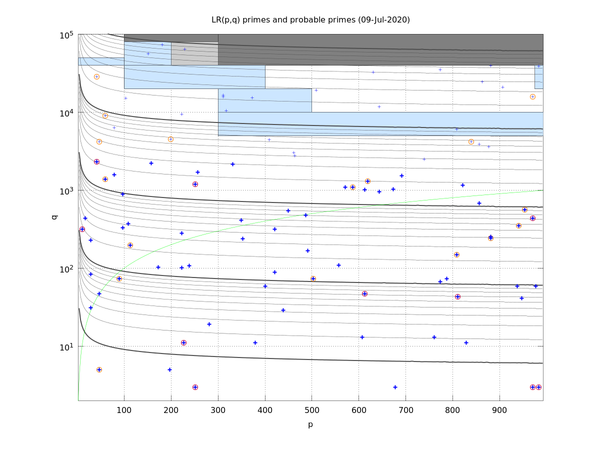Light gray areas are currently being processed. Blue areas have been mostly processed by the LR team : Anna, Athina, Dimitris, Elias, Fotini, Giorgos, Keira, Kostas, Koulla, Marios, Neoklis, Patrice, Theodora, Vicky, Xrisa. Thanks to all of them !

LR_data.pdf : known (probable) prime values LR(p,q) with p<1000

Numerical data

Tau_0001000000.zip (1.4 Mb): tau(p) for all primes p < 10^6
Tau_0010000000.zip (13.4 Mb): tau(p) for all primes p < 10^7

Publications

N. Lygeros & O. Rozier, Odd prime values of the Ramanujan tau function, Ramanujan Journal, 10.1007/s11139-012-9420-8 (2013)
N. Lygeros & O. Rozier, A new solution to the equation τ(p) ≡ 0 (mod p), Journal of Integer Sequences 13, article 10.7.4 (2010)